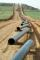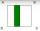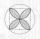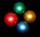# Flowerbed

Family cultivated tulips on a square flower bed of 6 meters. Later they added the square terrace with a side of 7 meters to their house. One vertex of the terrace lay exactly in the middle of a tulip bed, and one side of the terrace divided the side of the tulip bed in a ratio of 1: 5.
In what proportion did the other side of the terrace split the other side of the flower bed? How many square meters of terraces have their tulips shrunk?

Result

M = (Correct answer is: 1:5)S =  9 m2

#### Solution:Leave us a comment of example and its solution (i.e. if it is still somewhat unclear...):Be the first to comment!#### To solve this example are needed these knowledge from mathematics:

Pythagorean theorem is the base for the right triangle calculator.

## Next similar examples:

1. PipelineHow much percent has changed (reduced) area of pipe cross-section, if circular shape changed to square with same perimeter?
2. Folded squareABCD is a square. The square is folded on the midpoint of AB and A is folded onto the fold, creating a shaded region. The perimiter of the shaded figure is 75. Find the area of square ABCD
3. QuatrefoilCalculate area of the quatrefoil which is inscribed in a square with side 6 cm.
4. WindbreakA tree at a height of 3 meters broke in the windbreak. Its peak fell 4.5 m from the tree. How tall was the tree?The double ladder is 8.5m long. It is built so that its lower ends are 3.5 meters apart. How high does the upper end of the ladder reach?The double ladder shoulders should be 3 meters long. What height will the upper top of the ladder reach if the lower ends are 1.8 meters apart?
7. Broken treeThe tree was 35 meters high. The tree broke at a height of 10 m above the ground. Top but does not fall off it refuted on the ground. How far from the base of the tree lay its peak?
8. Theorem proveWe want to prove the sentence: If the natural number n is divisible by six, then n is divisible by three. From what assumption we started?
9. Rectangular trapezoidCalculate the content of a rectangular trapezoid with a right angle at the point A and if |AC| = 4 cm, |BC| = 3 cm and the diagonal AC is perpendicular to the side BC.
10. Truncated cone 5The height of a cone 7 cm and the length of side is 10 cm and the lower radius is 3cm. What could the possible answer for the upper radius of truncated cone?
11. DistanceWha is the distance between the origin and the point (18; 22)?
12. Is right triangleDecide if the triangle XYZ is rectangular: x = 4 m, y = 6 m, z = 4 m
13. 4s pyramidRegular tetrahedral pyramid has a base edge a=17 and collaterally edge length b=32. What is its height?
14. BW-BS ballsAdam has a full box of balls that are large or small, black or white. Ratio of large and small balls is 5:3. Within the large balls the ratio of the black to white is 1:2 and between small balls the ratio of the black to white is 1:8 What is the ratio of.
15. Right trianglesHow many right triangles we can construct from line segments 3,4,5,6,8,10,12,13,15,17 cm long? (Do not forget to the triangle inequality).In six baskets, the seller has fruit. In individual baskets, there are only apples or just pears with the following number of fruits: 5,6,12,14,23 and 29. "If I sell this basket," the salesman thinks, "then I will have just as many apples as a pear." WhichThe radius of the base of a right circular cone is 14 inches and it's height 18 inches. What is the slant height?